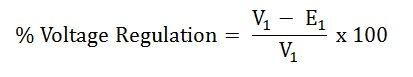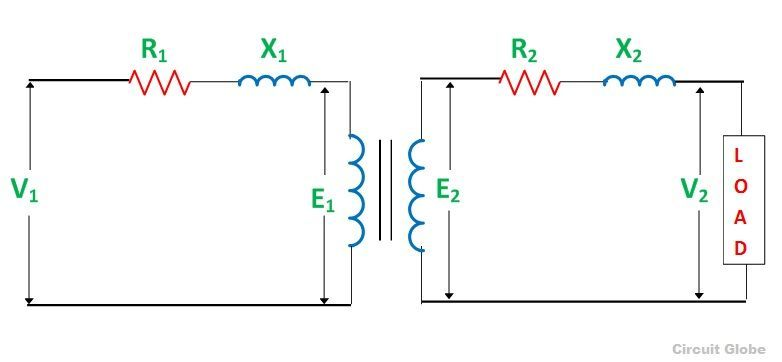# Voltage Regulation of a Transformer

Definition: The voltage regulation is defined as the change in the magnitude of receiving and sending voltage of the transformer. The voltage regulation determines the ability of the transformer to provide the constant voltage for variable loads.

When the transformer is loaded with continuous supply voltage, the terminal voltage of the transformer varies. The variation of voltage depends on the load and its power factor.

Mathematically, the voltage regulation is represented as:where,
E2 – secondary terminal voltage at no load
V2 – secondary terminal voltage at full load

The voltage regulation by considering the primary terminal voltage of the transformer is expressed as,Let us understand the voltage regulation by taking an example explained below:

If the secondary terminals of the transformer are open-circuited or no load is connected to the secondary terminals,  the no-load current flows through it.

If the no current flows through the secondary terminals of the transformer, the voltage drops across their resistive and reactive load become zero. The voltage drop across the primary side of the transformer is negligible.

If the transformer is fully loaded, i.e., the load is connected to their secondary terminal,  the voltage drops appear across it. The value of the voltage regulation should always be less for the better performance of the transformer.From the circuit diagram shown above, the following conclusions are made

• The primary voltage of the transformer is always greater than the induced emf on the primary side. V1>E1
• The secondary terminal voltage at no load is always greater than the voltage at full load condition. E2>V2

By considering the above circuit diagram, the following equations are drawnThe approximate expression for the no-load secondary voltage for the different types of the load is

In this way, we define the voltage regulation of the transformer.